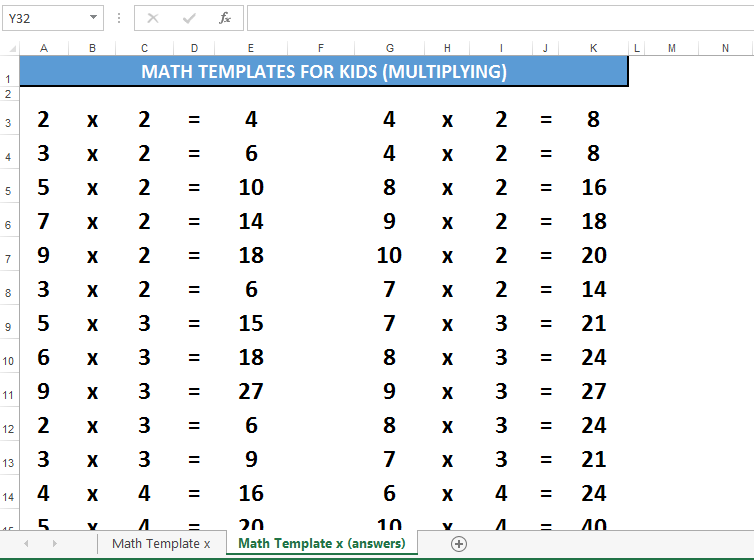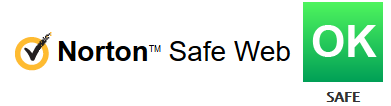# Math for Kids template multiplying numbers

Save, fill-In The Blanks, Print, Done!Click on image to zoom / Click button below to see more images

• This Document Has Been Certified by a Professional
• 100% customizable
• Language: English

ABT template rating: 7

Malware- and virusfree. Scanned by:Do you need a Multiplication Worksheet template for your children? What is the strategy you use to teach your kids multiplication? Download our template now and use it for your children to practice their math skills. These worksheets are designed to challenge children and help them progress in their math studies.

Teaching multiplication to kids can be an engaging and rewarding experience. Here are some effective strategies and tips for teaching multiplication to children:

• Begin with the concept of multiplication as repeated addition. Explain that multiplication is a way of adding the same number multiple times. For example, 3 × 4 is the same as adding 3 four times (3 + 3 + 3 + 3).
2. Use Visual Aids:
• Visual aids, such as arrays, grids, or objects like blocks or coins, can help children grasp multiplication concepts. Show them how to create equal groups to represent multiplication.
3. Multiplication Tables:
• Introduce multiplication tables or charts. Focus on one number at a time, starting with the times tables for 2, 5, and 10, as they tend to be easier for kids to grasp initially.
4. Skip Counting:
• Teach skip counting, which is counting by a specific number (e.g., counting by twos, fives, or tens). This helps children understand the patterns in multiplication.
5. Use Manipulatives:
• Hands-on materials like counters, beads, or drawing pictures can help kids visualize and understand multiplication. For instance, show them how to represent 4 × 3 using four groups of three objects.
6. Engage in Practical Activities:
• Incorporate multiplication into everyday activities. For example, ask your child to calculate the total number of items when you buy multiples of a product at the store.
7. Flashcards:
• Use multiplication flashcards to practice and reinforce multiplication facts. Flashcards can be a quick and effective way to build fluency.
8. Relate to Division:
• Explain the connection between multiplication and division. Emphasize that multiplication is the inverse operation of division.

Remember that learning multiplication is a gradual process, and it's normal for children to take time to become proficient. Be encouraging, and create a positive learning environment that fosters their curiosity and enthusiasm for math.

Strategy: The Multiplication Chart Method

1. Introduction to Multiplication: Start by explaining multiplication as repeated addition.
2. Introduce the Multiplication Chart: Use a blank multiplication chart to visualize multiplication concepts.
3. Fill in the Chart: Together, fill in the chart for a selected times table (e.g., 2 times table) by calculating and writing the products.
4. Visualize Patterns: Help children see patterns in the completed chart, such as numbers ending in 0, 2, 4, 6, or 8, and diagonal patterns with equal numbers.

This strategy uses a visual aid (the multiplication chart) to reinforce multiplication concepts and patterns, making learning multiplication engaging and effective.

Using this "how to start doing math" template guarantees your family a funny learning experience that stimulates the brain and memory of your child(ren) and yourself!

DISCLAIMER
Nothing on this site shall be considered legal advice and no attorney-client relationship is established.## Christmas Templates

### Latest templates

The trick is in what one emphasizes. We either make ourselves miserable, or we make ourselves strong. The amount of work is the same. – | Carlos Castaneda